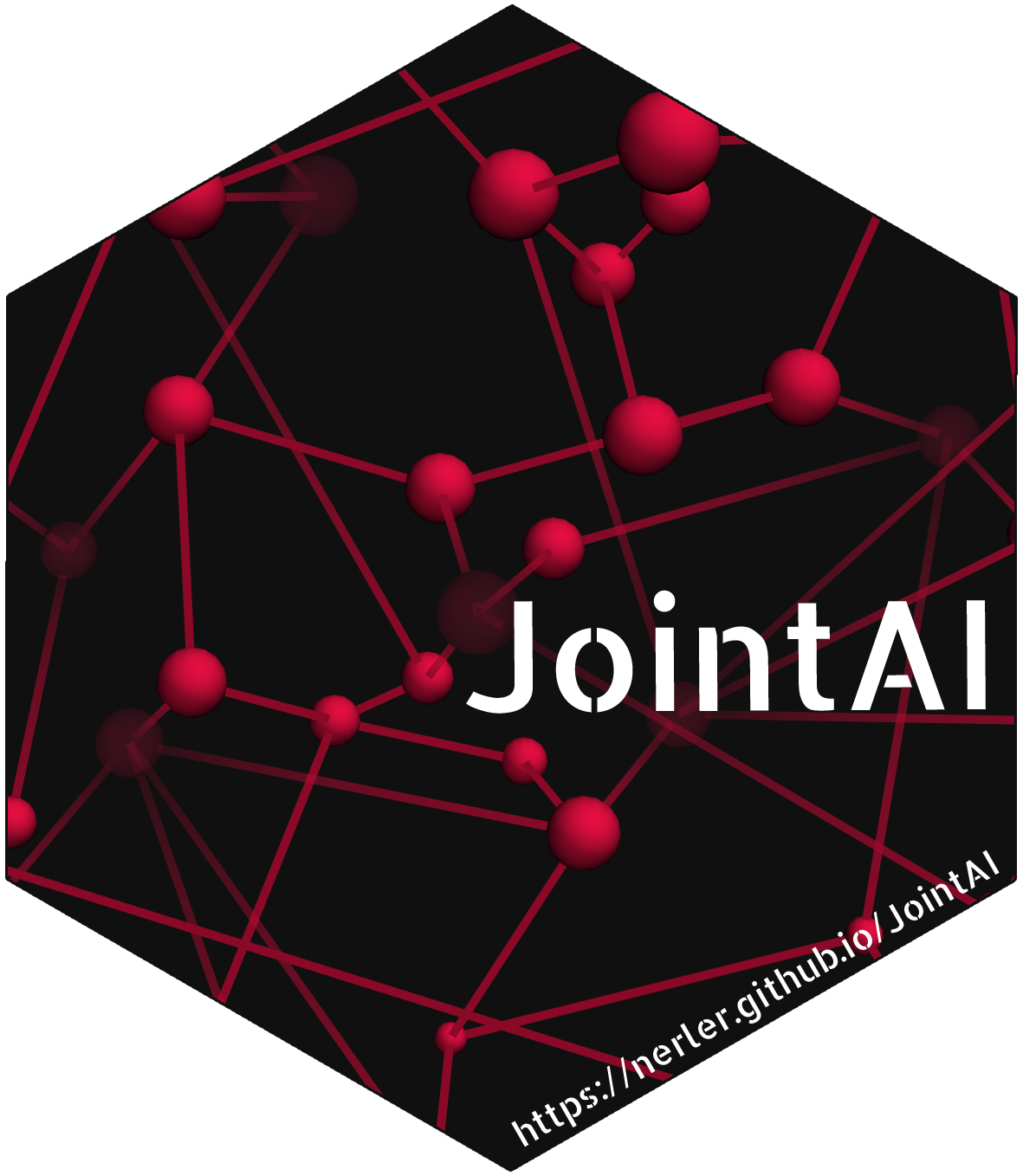# Joint Analysis and Imputation of Incomplete Data in RJointAI is a software package contributed to the Comprehensive R Archive Network (CRAN). It provides functionality to fit

• generalized linear regressionmodels
• (ordinal) cumulative logit regression models
• generalized linear mixed models (for multi-level data)
• (ordinal) cumulative logit mixed models
• parametric (Weibull) survival models
• Cox proportional hazards survival models

on incomplete (covariate) data using the Bayesian framework.

As far as possible, the specification of the main functions is analogue to the specification of their complete data versions from base R and the packages ordinal, nlme and survival.

JointAI performs some preprocessing of the data and creates a “JAGS” model, which will then automatically be passed to JAGS with the help of the R package rjags.

JointAI has its own website, it can be downloaded from CRAN or GitHub, and it is part of the CRAN Task View: Missing Data.

### Special features

Some features include parallel computation, shrinkage of regression coefficiens via ridge penalties and the option to use user-specified hyperparameters.

### Results

Results can not only be summarized and printed, but also visualized using traceplots or density plots.

### Prediction

It is also possible to obtain predicted values (and corresponding intervals) from JointAI models.

### Evaluation of convergence and precision

Two criteria for evaluation of convergence and precision of the posterior estimate are available:
The Gelman-Rubin criterion (‘potential scale reduction factor’) for convergence1, and the Monte Carlo error, specifically the ratio of the Monte Carlo error to the parameter’s standard deviation.

### Extraction and visualization of imputed values

Imputed data can be extracted, as multipe imputed datasets for further analyses in R,and directly exported to SPSS. Once extracted, the distribution of the imputed values can be compared visually to the distribution of the observed data.

### Visualization of incomplete data

To learn about the data at hand, and make better modelling choices, functions that calculate and plot the missing data pattern and the distribution of each of the variables in the dataset, are provided.

## Vignettes

The package comes with the following vignettes, which give a more detailed explanation and demonstration of how to use JointAI:

### Minimal Example

A minimal example demonstrating the use of the functions lm_imp(), summary(), traceplot() and densplot().

### Visualizing Incomplete Data:

Demonstrations of the options in the functions plot_all() (plotting histograms and barplots for all variables in the data) and md_pattern() (plotting or printing the missing data pattern).

### Model Specification:

Explanation and demonstration of all parameters that are required or optional to specify the model structure in the main anlysis functions. Among others, the additional functions parameters(), list_models(), get_models() and set_refcat() are used.

### Parameter Selection:

Examples on how to select the parameters/variables/nodes to follow using the argument monitor_params and the parameters/variables/nodes displayed in the functions summary(), traceplot(), densplot() or when using GR_crit() or MC_error().

### MCMC Settings:

Examples demonstrating how to set the arguments controlling settings of the MCMC sampling, i.e., n.adapt, n.iter, n.chains, thin, inits.

### After Fitting:

Examples on the use of functions to be applied after the model has been fitted, including traceplot(), densplot(), summary(), GR_crit(), MC_error(), predict(), predDF() and get_MIdat().

1. Gelman, A and Rubin, DB (1992) Inference from iterative simulation using multiple sequences, Statistical Science, 7, 457-511.
Brooks, SP. and Gelman, A. (1998) General methods for monitoring convergence of iterative simulations. Journal of Computational and Graphical Statistics, 7, 434-455. ↩︎